Get instant live expert help with Excel or Google Sheets“My Excelchat expert helped me in less than 20 minutes, saving me what would have been 5 hours of work!”

#### Post your problem and you’ll get expert help in seconds.

Your message must be at least 40 characters
Our professional experts are available now. Your privacy is guaranteed.

# Random Number Generator in Excel and Google Sheets

In this tutorial, we will learn how to use RAND in Excel and to get a Google Sheets random number generator. This step by step tutorial will assist all levels of Excel users to get the random decimal or non-decimal number in Excel and Google Sheets.

Here are the topics that will be covered:

• Get Excel Random Number Between Decimals with RAND Function
• RANDBETWEEN Decimal Numbers in Excel
• Use RANDBETWEEN Function to Get Random Non-Decimal Number
• Google Sheets Random Number Generator
• Google Sheets Random Decimal Number

## Get Excel Random Number Between Decimals with RAND Function

If we want to have a random number between decimals in Excel, we should use the RAND function. This function generates a random decimal number between 0 and 1. There are no arguments for this function. We just have to write formula `=RAND ()`.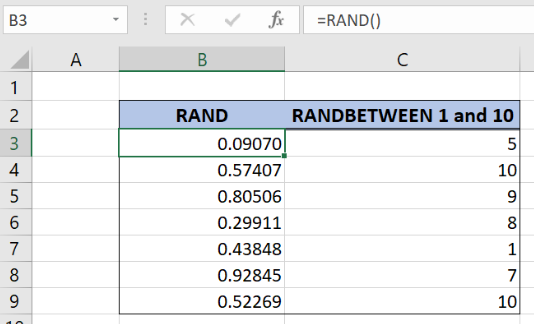Figure 1. How to use RAND in excel

• Select the cell B3 and write the formula: `=RAND()`
• Press enter
• Drag the formula down to the other cells in the column by clicking and dragging the little “+” icon at the bottom-right of the cell.

Note that this function is volatile, and whenever we click on another cell random numbers will change. To prevent this we can copy random numbers between decimals with Ctrl+C and paste them as values (right mouse click and Paste Options: Values).

## RANDBETWEEN Decimal Numbers in Excel

Logic is the same if we want to RANDBETWEEN decimal numbers in Excel that are in the other number range than 0 and 1. In our example, we want to get a random decimal number between 1 and 10. Select the cell D3 and insert the formula: =1+9*RAND(). Copy the formula down by dragging icon “+” of the cell, as explained in the example above.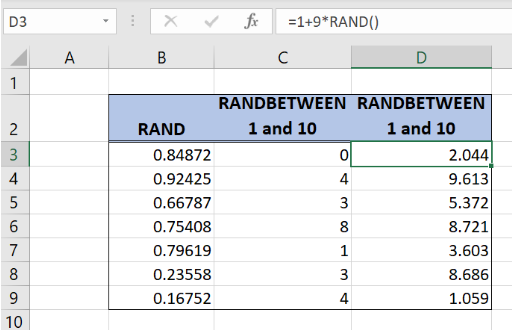Figure 2. How to use RAND in Excel to get a random decimal number

Because we want a decimal number, top number 10 should be subtracted with number 1 and multiplied with RAND function result that is always a decimal number between 0 and 1.

When RAND function result is 0 formula output is number 1, and when the RAND function result is number 1 formula output is number 10. As a result, the final number is a random decimal number between 1 and 10.

## Use RANDBETWEEN Function to Get Random Non-Decimal Number

To get a random non-decimal number between the top and bottom numbers we can use RANDBETWEEN function. Formula syntax is easy, it has a bottom and top range numbers. In our example, we want to generate random non-decimal numbers between 1 and 10.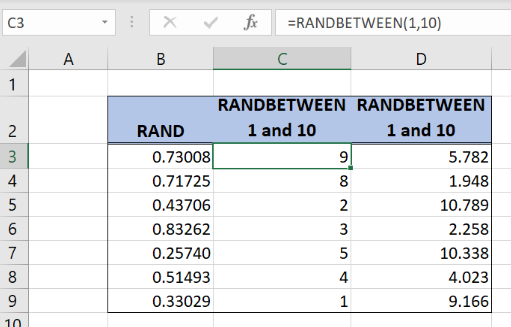Figure 3. Get random non-decimal numbers with RANDBETWEEN function

• Select the cell C3 and write the formula: `=RANDBETWEEN(1,10)`
• Press enter
• Drag the formula down to the other cells in the column by clicking and dragging the little “+” icon at the bottom-right of the cell.

## Google Sheets Random Number Generator

To get a random number generator in Google sheets between 0 and 1 we can use RAND function. Insert RAND formula in the cell B3: =RAND() and copy the formula down by dragging the icon “+” in the bottom-right of the cell.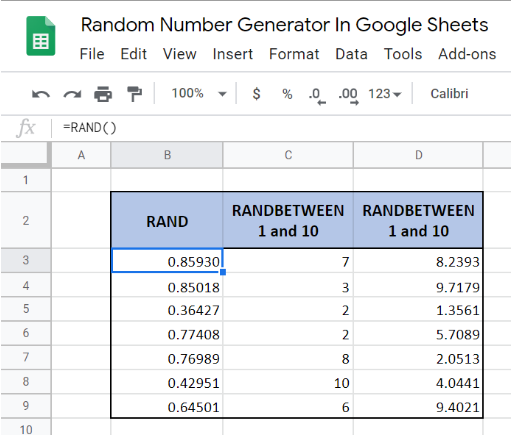Figure 4. Random number generator Google Sheets

As in Excel, RANDBETWEEN function can be used to get random non-decimal numbers between the defined number range. In our example, we want to get a random non-decimal number between 1 and 10.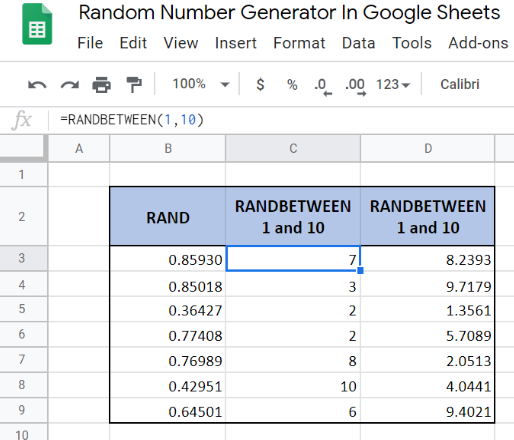Figure 5. Google Sheets random non-decimal number

• Select the cell C3 and write the formula: `=RANDBETWEEN(1,10)`
• Press enter
• Drag the formula down to the other cells in the column by clicking and dragging the little “+” icon at the bottom-right of the cell.

## Google Sheets Random Decimal Number

Like in Excel, we can use the RAND function to generate a random number in Google Sheets that is decimal. We want to get the decimal number between 1 and 10. To get this we should use the formula: `=1+9*RAND()`. The formula is the same as in the Excel example.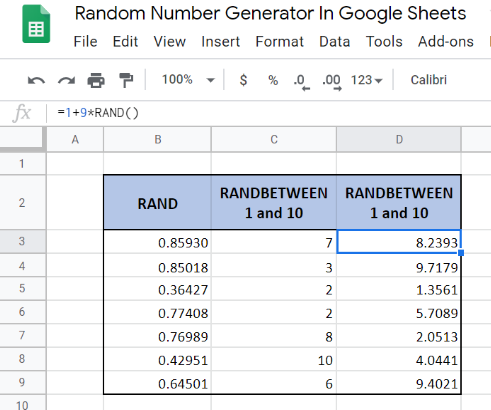Figure 6. Google Sheets random decimal number

• Select the cell D3 and write the formula: `=1+9*RAND()`
• Press enter
• Drag the formula down to the other cells in the column by clicking and dragging the little “+” icon at the bottom-right of the cell.

Find out more about RAND function and how to make a random pairing generator on this link: https://www.got-it.ai/solutions/excel-chat/excel-tutorial/random/learn-how-to-make-a-random-pairing-generator-in-excel

Most of the time, the problem you will need to solve will be more complex than a simple application of a formula or function. If you want to save hours of research and frustration, try our live Excelchat service! Our Excel Experts are available 24/7 to answer any Excel question you may have. We guarantee a connection within 30 seconds and a customized solution within 20 minutes.

Solution examplesI'm Apply a =RANDBETWEEN formula in one cells want to apply Formula to entire column and run formula when i hit the entire on the particular cell
Solved by M. S. in 20 minsHelp! In a coin toss I have a 1 in 2 (fifty-fifty) chance of getting heads or tails. I need a formula to determine my chance of getting 4 heads in a row or 4 tails in a row out of 10 coin tosses. If I get 4 in a row I win. What formula can I use to determine my chances of winning if I repeat the 10 tosses for hundreds or thousands of times? I get as far as =RANDBETWEEN(0,1) but I have not been able to figure out what formula to use to determine if there are 4 in a row in a group of 10 tosses, times thousands of groups with 10 tosses in each group. Thank-you for any help you can provide.
Solved by V. F. in 51 minsI have 4 column. when i fill the first 3 column. in 4th column i need a unique id number and i generate unique id number using RANDBETWEEN formula [ =CHAR(RANDBETWEEN(65,90))&CHAR(RANDBETWEEN(65,90))&RANDBETWEEN(1000,9900) ] but i want ..... when i'm filling the 3 column after that 4th column run this formula and generate unique id automatically
Solved by X. Q. in 20 minswhen i fill column A and B then C column generate Unique ID using RandBetween Formula {=CHAR(RANDBETWEEN(65,90))&CHAR(RANDBETWEEN(65,90))&RANDBETWEEN(1000,9900)} ...
Solved by S. Q. in 20 mins